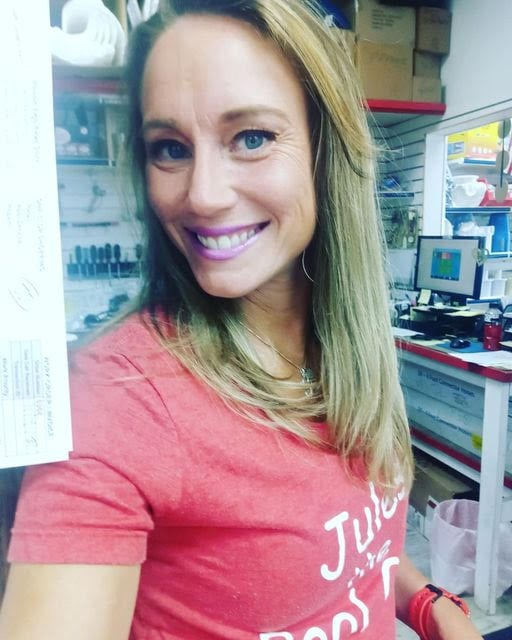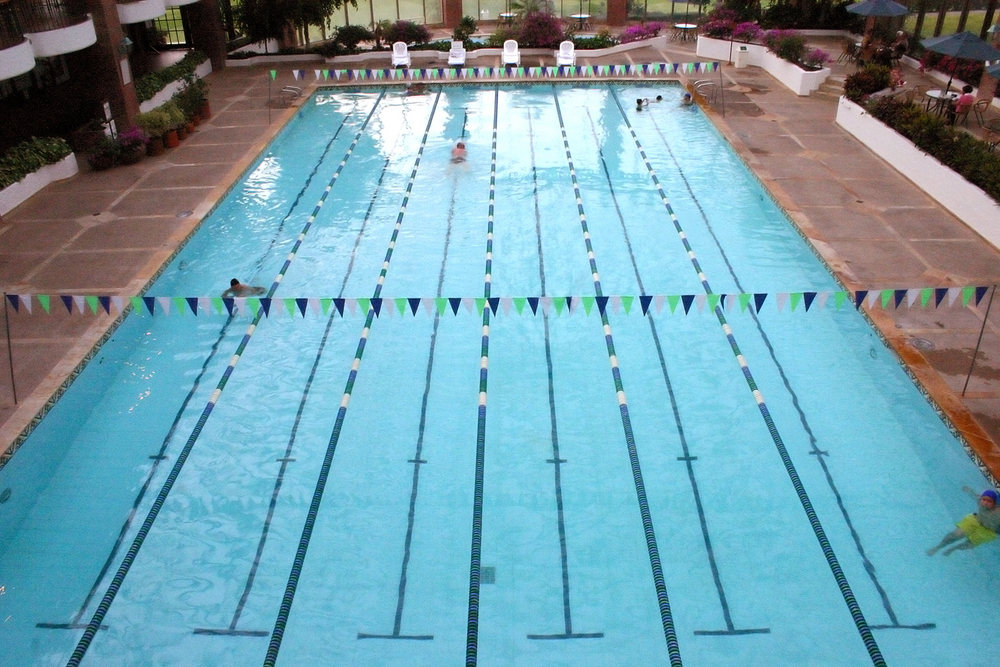855-742-7542

# Why Being Able to Calculate the Volume of a Commercial or Public Swimming Pool is ImportantPublished on Mar 18, 2022We have to factor in a lot of things when taking care of our commercial pools. Equipment and water chemistry are two big ones. But without knowing how many gallons of water our swimming pool has, we cannot make accurate decisions and perform the proper treatment.

## Plumbing Size

A builder must determine what plumbing size is needed when constructing a new pool build.  They cannot make this determination without knowing the size of the pool and how many gallons of water it will eventually hold. Proper plumbing size is crucial for proper circulation, flow rate and turnover rate.

## Equipment Size

Whether adding equipment for the first time or replacing existing equipment, we have to now take into consideration the plumbing size, the size of the pool, the desired turnover rate, and again, how many gallons of water are in the swimming pool.

For example, you wouldn’t want to put a 2 hp pump on a pool with inch and a half plumbing.  For variable speed pumps, based on the gallons per hour, a minimum of a 60 square foot filter is recommended.

## Chemical Dosing

This part is super important as pool operators because this is the part we are in charge of.  Typically, the pools we manage are already built and have existing equipment. We are in charge of the daily testing and dosing of the swimming pool chemicals. The Pool Shark H20 App, in which you are not only keeping logs but getting your dosing instructions, requires the gallons of the pool to properly execute dosing instructions. There are formulas that we discuss below to determine gallonage. You can figure it out with a few simple formulas.

## Square or Rectangular Pools

The easy formula for finding out how many gallons are in a square or rectangular pool is Length x width x average depth x 7.5. The average depth is measured by taking the deepest depth of the pool and the shallowest point of the pool and adding them together and then dividing it by 2.  For example, If you had a pool that was 7 feet at the deep end and 3 feet at the shallow end you would add 7 + 3 = 10.  10 divided by 2 = 5.  So in this example, this pool would have an average depth of 5 ft. The reason we multiply it by 7.5 is that there are 7.5 gallons for each cubic foot. So let’s say we have a pool that is 10 ft by 20 ft and an average depth of 5. We would do 20 x 10 x 5 x 7.5= 7,500 gallons.

If the pool has a severe slope, where most of it is shallow and then suddenly drops off to a deep end, this gives you a different average depth. In this case, you may want to divide the pool into two different sections, use the formula for each section and then add them together.

## Round Pools

The formula for circular pools is pi (3.14) x radius squared x average depth.  A radius is equal to half of the diameter.  What you will want to do is measure the widest part of the circle, and then just divide it by 2. When we “square” a number it just means to multiply that number by 2.  So if we had a pool that was 10 ft wide, we would get a radius of 5. Let’s just say our average depth of this pool is also 5. The formula would look like this, 3.14 x 5 x5 = 392.5.  Always round up to the nearest gallon.

When measuring a spa, sometimes we need to divide it into more than 2 sections due to the benches. Once again, add the sections together and that will give you your gallons

## Free Form Pools

This is more expected of a residential versus a commercial pool, but you never know. You’ll have to break each section into its own individual sections. Then use the formula for circle or rectangle. This will depend on the “shapes” of the areas you are measuring. Then once again, add the sections together.

## Why This is So Important in Chemical Dosing

Knowing how much water is in the pool will ensure you are not over, or under, dosing. Underdosing you won’t get the desired change. If talking about sanitizer, too little puts your bathers at risk. Adding too much of a chemical can also skew your desired change. Depending on the chemical, you may even cause damage to the surface, the plumbing, the equipment, and possibly irritate the bathers. So, step 1 of utilizing your Pool Shark H20 App is to obtain the proper gallonage in your swimming pool. See you poolside!Author

## The benefits of implementing Pool Shark include:

• Substantial ROI
• Better Water Conditions
• Improved Guest & Customer Satisfaction
• Plug and Play Ease of Use
• Stronger Risk Management & Reduced Liability
• Guides and Practices